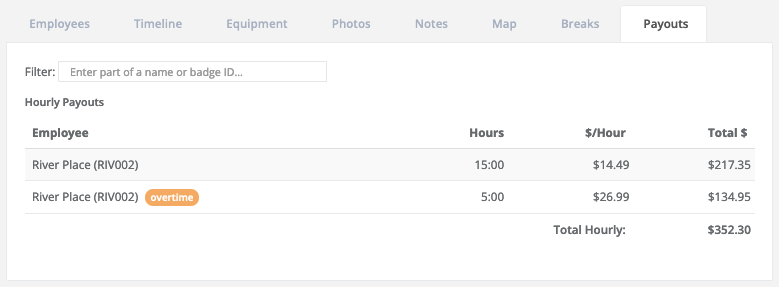# How does FieldClock calculate the Regular Rate of Pay (RRoP)?

### Formula

For a given Job, the Regular Rate of Pay is equal to the Total Pay of all previous Jobs (including OT) that the Employee worked plus the Base Pay for the current Job, divided by the Total Hours worked (including OT) for a given workweek.

• (Total Pay of all previous Jobs + Base Pay of current Job) / Total Hours worked

#### What does 'Base Pay' mean exactly?

Let's look at the following example:River's Total Pay here is \$352.30. His Base Pay, however, is different. In this example, it would be \$289.80 (20 hours x \$14.49). In other words, Base Pay is what the pay would be if there was no OT or DT.

### Calculating the Regular Rate of Pay

Let's assume the following, still using the above example:

• River's Wage Program states that he should earn OT after 55 hours in a single workweek
• River worked two Jobs before the one above
• Job 1, River worked 20 hours at \$14.49 for a Total Pay of \$289.80
• Job 2, River earned \$500 in piece pay

Regular Rate of Pay = \$289.80 (Job 1) + \$500 (Job 2) + \$289.80 (Job 3, using Base Pay) = \$17.99

### Calculating the overtime hourly rate

Now that we have the above Job's Regular Rate of Pay (\$17.99), we can simply multiple that by your multiplier that's set in your Wage Program, which in this case is 1.5x.

\$17.99 x 1.5 = \$26.99